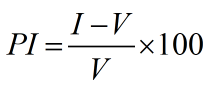Request a Tool

Percentage Increase Calculator

Percentage Increase Calculator is use to calculate increase percentage and its difference.

Increase
0
Difference
0

Formula• PI = Percentage increase
• V = Value
• I = Increase value

Defination / Uses

The difference between a final value and an initial value, presented as a percentage of the starting value, is the percent increase between two values.

If you need to compute an increase from one value to another as a percentage of the original amount, the percentage increase calculator might help. It may be helpful to understand how to compute percent increase using the percent increase formula before using this calculator. These principles will be explained in further depth in the following sections. The concept of % increase refers to the amount of change from the starting point to the end point in terms of 100 parts of the starting point. If you divide the original value into 100 parts, a 5% increase means that the value has increased by an additional 5 parts.